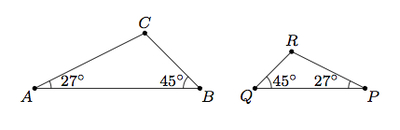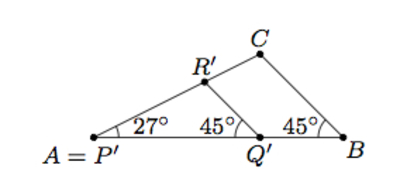# Similar Triangles II

Alignments to Content Standards: 8.G.A.5

Triangles $ABC$ and $PQR$ below share two pairs of congruent angles as marked:1. Explain, using dilations, translations, reflections, and/or rotations, why $\triangle PQR$ is similar to $\triangle ABC$.
2. Are angles $C$ and $R$ congruent?
3. Can you show the similarity in part a without using a reflection? What about without using a dilation? Explain.
4. Suppose $DEF$ and $KLM$ are two triangles with $m(\angle D) = m(\angle K)$ and $m(\angle E) = m(\angle L)$. Are triangles $DEF$ and $KLM$ similar?

## IM Commentary

The goal of the task is to provide an informal argument for the AA criterion for triangle similarity, appropriate for an 8th grade audience. The solution provided indicates the level of rigor expected in the argument for part (a). A more formal argument detailing which reflections, rotations, and translations are needed to superimpose $\angle P$ on $\angle A$ is appropriate for a high school task about the AA criterion (G.SRT.3): see https://www.illustrativemathematics.org/content-standards/tasks/1422. The solution to part (a) does not detail the transformations needed to move $\angle P$ to $\angle A$.  If the points $A$, $B$, $P$ and $Q$ are collinear then this can be accomplished with a reflection over the perpendicular bisector of $\overline{AP}$. Computer software could greatly enhance this task, helping students experiment and visualize the impact of different transformations.

For part (b) of the question, students can use the similarity that they established in part (a) or they might use the fact that the sum of the angles in a triangle is always 180 degrees if they have already seen this result. Alternatively, using the picture in the solution, they could argue that $\overleftrightarrow{R'Q'}$ and $\overleftrightarrow{CB}$ are parallel. Line $\overleftrightarrow{AC}$ is a transversal for these parallel lines and from here students could argue that $\angle R'$ and $\angle C$ are congruent. Part (c) of the question asks students to reflect on the nature of translations, rotations, reflections, and dilations. Only a reflection changes the orientation of the plane, that is switches the notions of clockwise and counterclockwise, and so a reflection is needed to show this similarity. In the same way, only a dilation changes distances between points and so a dilation is also necessary to show the similarity. How and when the reflection and dilation are applied may of course vary: moreover, it is possible that multiple reflections and/or dilations are applied.

Finally for part (d), although no picture of the triangles $DEF$ and $KLM$ is given, the ideas used to construct the similarity in part (a) apply to any pair of similar triangles. The goal of this part of the task is to have students think about and articulate why the construction in part (a) can be generalized.

## Solution

1. We can first match up $\angle P$ exactly with $\angle A$: this will require a reflection and possibly also translations and/or rotations. This gives the picture below:The image of $\triangle PQR$ has been labeled $P^\prime Q^\prime R^\prime$. Because $m(\angle P) = m(\angle A) = 27$, we can align the two triangles as in the picture. In order to move $Q'$ to $B$ we can apply a dilation with center $A$. The scale factor for the dilation will need to be $\frac{|AB|}{\left|P^\prime Q^\prime\right|}$ in order to move $Q^\prime$ to $B$. Because $m(\angle P′Q′R′)=m(\angle ABC)$, $\triangle P'Q'R'$ coincides completely with $\triangle ABC$ after the dilation.  The dilation and rigid motions described show that $\triangle ABC$ and $\triangle PQR$ are similar.

2. Angles $C$ and $R$ are congruent. Because the dilation and rigid motions in part (a) do not change angle measures, angle $P'Q'R'$ will match up with angle $B$, and $\overline{R'Q'}$ will align precisely with $\overline{CB}$. Angle $R$ and angle $C$ will also coincide which means they are congruent.

3. It is not possible to show the similarity without using a reflection. In the argument for part (a), placing angle $P$ on top of angle $A$ requires a reflection. This is because going through the vertices of $\triangle ABC$ we move in a counterclockwise direction while going through the vertices of $\triangle PQR$ we move in a clockwise direction. Only reflections change the orientation of clockwise and counterclockwise.

It is also not possible to establish the similarity of triangles without a dilation. The triangles are of different size and only dilations change lengths of line segments.

4. The method used in part (a) will work for any pair of triangles $DEF$ and $KLM$ sharing two congruent angles. As we did with $\triangle ABC$ and $\triangle PQR$, we can first move $\triangle KLM$ so that $\angle K$ matches up with $\angle D$ (this may require a reflection or might be accomplished with a translation and/or rotation). At this point we can dilate the plane about the point $D$. Labelling the image of $\triangle KLM$ as $K'L'M'$, if we dilate by a factor of $\frac{|DE|}{\left|K'L'\right|}$ this will map $L$ to $E$. Because $m(\angle L) = m(\angle E)$, this shows that triangles $DEF$ and $KLM$ are similar.